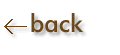Interpretation of Graphs:

DIRECT  RELATIONSHIP                                                    INVERSE  RELATIONSHIP

 If two variables are directly proportional, then as one variable (x) increases, the other changes proportionally.  A graph of this function will produce a straight line.  The equation is y = kx. If two variables are inversely proportional, it means that as one variable (x) increases, the other variable (y) decreases.  A graph of this function will produce a hyberbolic curve.  The equation is xy = k, or y = k(1/x)